# USING PYTHON LANGUAGE. QUESTION I: Write a program that displays the following table: a a^2 a...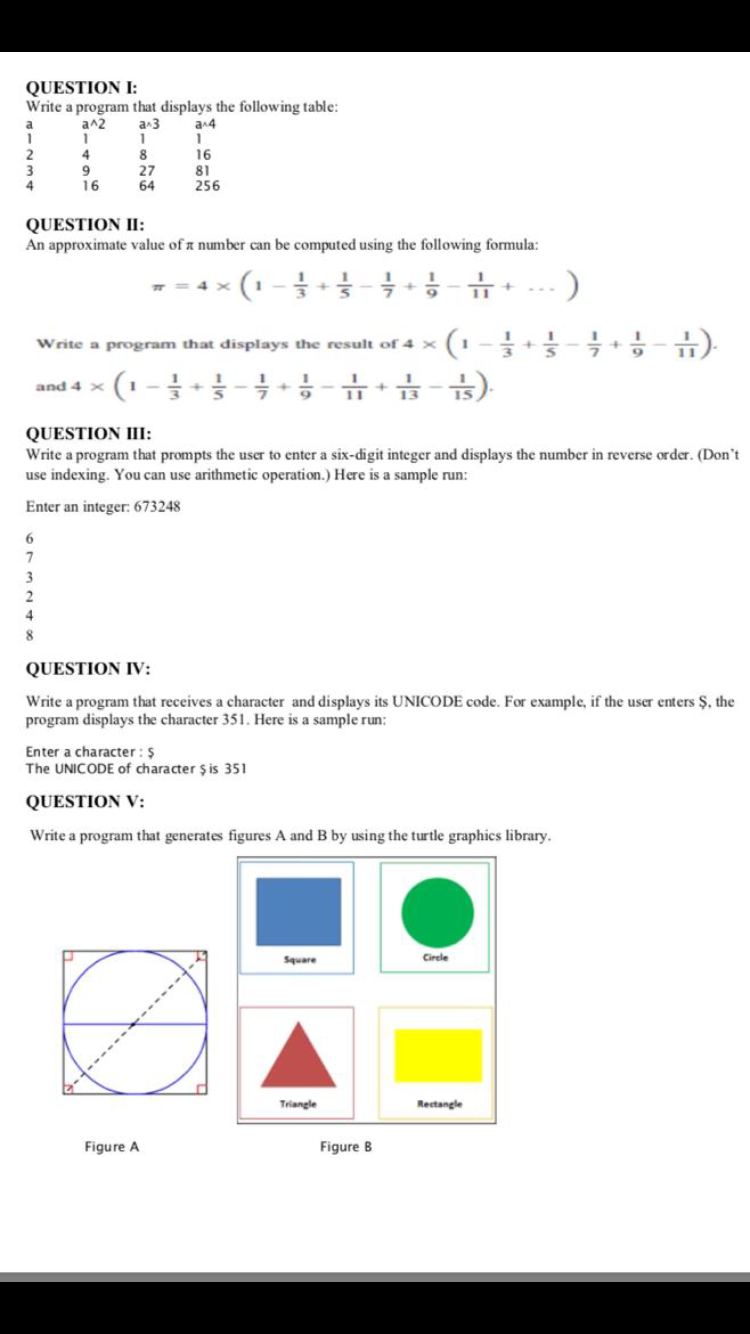USING PYTHON LANGUAGE.

QUESTION I: Write a program that displays the following table: a a^2 a 3 a14 1 1 1 1 2 4 8 16 3 9 27 81 4 16 64 256 QUESTION II: An approximate value of a number can be computed using the following formula: 7 ) Write a program that displays the result of 4 X + 7 • H) and 4 X - 를 7 tis). 9 QUESTION III: Write a program that prompts the user to enter a six-digit integer and displays the number in reverse order. (Don't use indexing. You can use arithmetic operation.) Here is a sample run: Enter an integer: 673248 6 7 3 2 4 8 QUESTION IV: Write a program that receives a character and displays its UNICODE code. For example, if the user enters \$, the program displays the character 351. Here is a sample run: Enter a character: \$ The UNICODE of character is 351 QUESTION V: Write a program that generates figures A and B by using the turtle graphics library. Square Circle e Triangle Rectangle Figure A Figure B

1.

``````print("a\ta^2\t^a^3\ta^4")
for i in range(1,5):
print(i,"\t",i*i,"\t",i*i*i,"\t",i*i*i*i)``````

2.

``````print("approximate value of pie using 4*(1-1/3+1/5-1/7+1/9-1/11) is : ", 4*(1-1/3+1/5-1/7+1/9-1/11) )
print("approximate value of pie using 4*(1-1/3+1/5-1/7+1/9-1/11+1/13-1/15) is : ", 4*(1-1/3+1/5-1/7+1/9-1/11+1/13-1/15) )
``````

3. Here, it is asked to return reverse order but in example it is shown in the normal order , but I have done coding for both reverse order and normal order

``````number = int(input("Enter a 6 digit number : "))

new_number = 0
print("numbers in reverse order")
while(number>0):
remainder = number%10
number = number//10
print(remainder)
new_number = new_number*10+remainder

print("numbers in original order")
while(new_number>0):
remainder = new_number%10
new_number = new_number//10
print(remainder)``````

4.

``````character = input("Enter a character")
print("UniCode Code for given character is : ",ord(character))``````

5.

``````import turtle
import math
skk = turtle.Turtle()
print(turtle.position())

for i in range(4):
skk.pencolor("red")
skk.forward(5)
skk.right(90)
skk.forward(5)
skk.right(90)
skk.forward(5)
skk.right(90)
skk.forward(5)
skk.right(90)
skk.pencolor("black")
skk.forward(100)
skk.right(90)

# for i in range()
skk.setpos(0,-50)
skk.right(90)
skk.pencolor("blue")
skk.circle(50)
skk.left(90)
skk.forward(100)
skk.pencolor("black")
skk.left(90)
skk.forward(50)
skk.left(135)
j = 0
while (j<35):
if(j%2 == 0):
skk.penup()
skk.forward(4)
else :
skk.pendown()
skk.forward(4)
j = j+1

turtle.done()``````

6.

``````import turtle
import math

skk = turtle.Turtle()

for i in range(4):
skk.forward(200)
skk.right(90)

skk.penup()
skk.forward(5)
skk.right(90)
skk.forward(5)
skk.left(90)
skk.pendown()
skk.pencolor("blue")
for i in range(4):
skk.forward(80)
skk.right(90)

skk.penup()
skk.forward(110)
skk.pendown()
skk.pencolor("green")
for i in range(4):
skk.forward(80)
skk.right(90)

skk.penup()
skk.right(90)
skk.forward(110)
skk.pendown()
skk.pencolor("yellow")
skk.left(90)
for i in range(4):
skk.forward(80)
skk.right(90)

skk.penup()
skk.right(180)
skk.forward(110)
skk.pendown()
skk.pencolor("red")
skk.left(180)
for i in range(4):
skk.forward(80)
skk.right(90)

skk.penup()
skk.forward(15)
skk.right(90)
skk.forward(60)
skk.pendown()
skk.fillcolor('red')
skk.begin_fill()
skk.left(90)
skk.forward(50)
skk.left(120)
skk.forward(50)
skk.left(120)
skk.forward(50)
skk.end_fill()

skk.penup()
skk.left(120)
skk.forward(105)
skk.pendown()
skk.pencolor('yellow')
skk.fillcolor('yellow')
skk.begin_fill()
skk.forward(60)
skk.left(90)
skk.forward(40)
skk.left(90)
skk.forward(60)
skk.left(90)
skk.forward(40)
skk.left(180)
skk.end_fill()

skk.penup()
skk.forward(130)
skk.right(90)
skk.forward(5)
skk.pendown()
skk.pencolor('green')
skk.fillcolor('green')
skk.begin_fill()
skk.right(90)
skk.circle(25)
skk.right(90)
skk.end_fill()

skk.penup()
skk.forward(55)
skk.left(90)
skk.forward(30)
skk.pendown()
skk.pencolor('blue')
skk.right(90)
skk.fillcolor('blue')
skk.begin_fill()
for i in range(4):
skk.forward(60)
skk.right(90)
skk.end_fill()``````

Just a request , Please don`t so much questions at one go , It`s difficult to answer all of them in just 2-3 hrs span . Better break half quantity and ask , it would help us also .
Hope the answers help, If any issue do comment !!

#### Earn Coin

Coins can be redeemed for fabulous gifts.

Similar Homework Help Questions
• ### write a java program that displays the following: Write a Java program that prompts the user...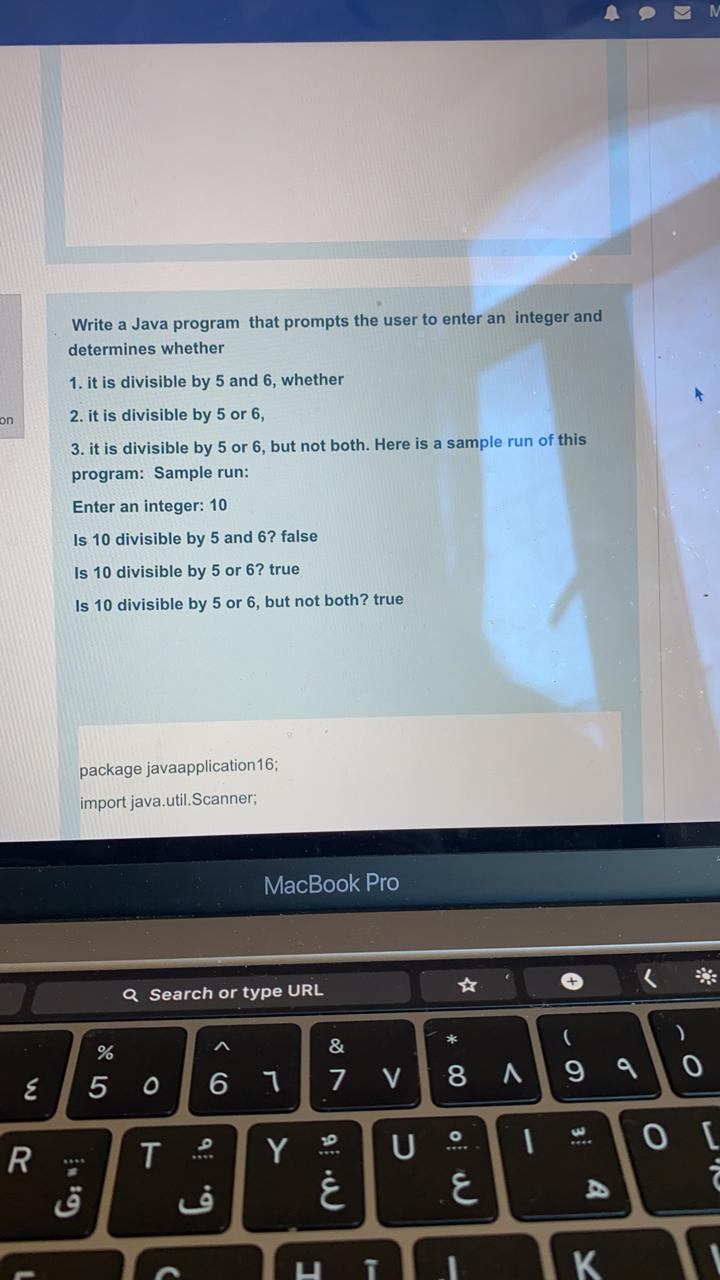write a java program that displays the following: Write a Java program that prompts the user to enter an integer and determines whether 1. it is divisible by 5 and 6, whether on 2. it is divisible by 5 or 6, 3. it is divisible by 5 or 6, but not both. Here is a sample run of this program: Sample run: Enter an integer: 10 Is 10 divisible by 5 and 6? false Is 10 divisible by 5 or...

• ### Q: 4.9 (Find the Unicode of a character) Write a program that receives a character and...

Q: 4.9 (Find the Unicode of a character) Write a program that receives a character and displays is Unicode. Here is a saple run: Enter a character: E < Enter The Unicode for the character E is 69. Interdaction to Java Programming, Brief Version. 10th Edition.

• ### Write a program that receives a character and displays its Unicode. using java please

Write a program that receives a character and displays its Unicode. using java please

• ### Please help with 3.12 and 3.14 Write a program that prompts the user to enter the...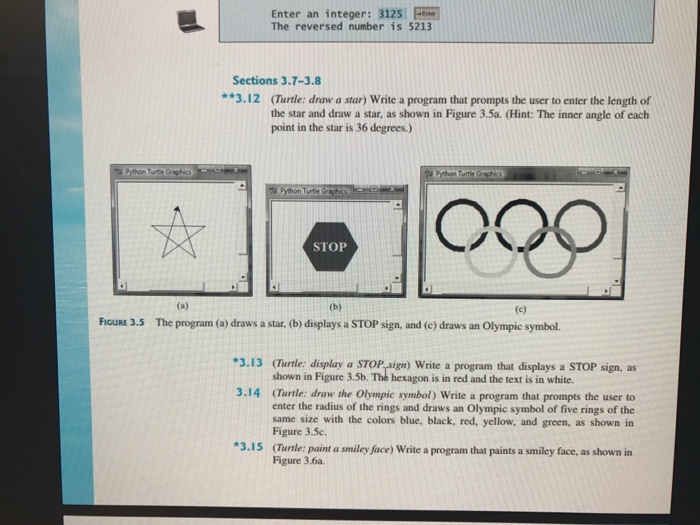Please help with 3.12 and 3.14 Write a program that prompts the user to enter the length of the star and draw a star, as shown in Figure 3.5a. (Turtle: display a STOP_sign) write a program that displays a STOP sign, as shown in Figure 3.5b. The hexagon is in red and the text is in white. (Turtle: draw the Olympic symbol) write a program that prompts the user to enter the radius of the rings and draws an Olympic...

• ### Write a C program that does the following: • Displays a menu (similar to what you...

Write a C program that does the following: • Displays a menu (similar to what you see in a Bank ATM machine) that prompts the user to enter a single character S or D or Q and then prints a shape of Square, Diamond (with selected height and selected symbol), or Quits if user entered Q. Apart from these 2 other shapes, add a new shape of your choice for any related character. • Program then prompts the user to...

• ### Part I: Code writing: 1. 3pts (Display matrix of numbers) Write a function that displays an...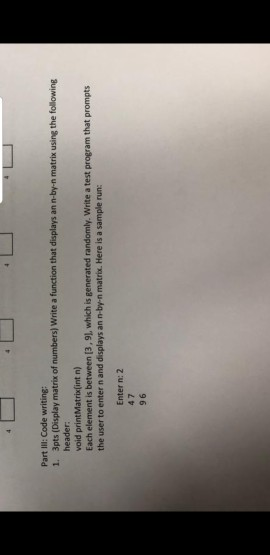Part I: Code writing: 1. 3pts (Display matrix of numbers) Write a function that displays an n-by-n matrix using the following header: void printMatrix(int n) Each element is between (3,9), which is generated randomly. Write a test program that prompts the user to enter n and displays an n-by-n matrix. Here is a sample run: Enter n: 2 47 96

• ### (Python) Please do NOT solve it by using special functions(like max min, divmod), I have to...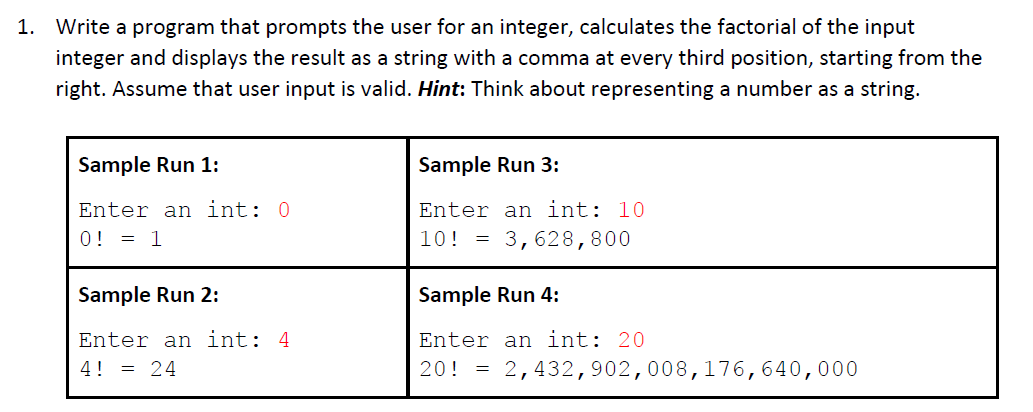(Python) Please do NOT solve it by using special functions(like max min, divmod), I have to ask the same questions again and again otherwise, thanks for your understanding. 1. Write a program that prompts the user for an integer, calculates the factorial of the input integer and displays the result as a string with a comma at every third position, starting from the right. Assume that user input is valid. Hint: Think about representing a number as a string. Sample...

• ### Python 3 **11.40 (Guess the capitals) Write a program that repeatedly prompts the user to enter...

Python 3 **11.40 (Guess the capitals) Write a program that repeatedly prompts the user to enter a capital for a state. Upon receiving the user input, the program reports whether the answer is correct. Assume that 50 states and their capitals are stored in a two- dimensional list, as shown in Figure 11.13. The program prompts the user to answer all the states’ capitals and displays the total correct count. The user’s answer is not case sensitive. Implement the program...

• ### need help Write a program that prompts the user for student grades and displays the highest...

need help Write a program that prompts the user for student grades and displays the highest and lowest grades in the class. The user should enter a character to stop providing values. example Enter as many student grades as you like. Enter a character to stop. The highest grade is: 92.0 The lowest grade is: 10.65 it needs to be in java

• ### Write a program that prompts the user to enter two characters and displays the major and status represented in the characters.

Write a program that prompts the user to enter two characters and displays the major and status represented in the characters. The first character indicates the major and the second is number character 1, 2, 3, 4, which indicates whether a student is a freshman, sophomore, junior, or senior. Suppose the following characters are used to denote the majors: M: MathematicsC: Computer Science T: Testing and Certification